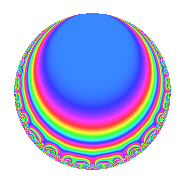# Properties

 Label 57.2.d.aLevel 57 Weight 2 Character orbit 57.d Analytic conductor 0.455 Analytic rank 0 Dimension 4 CM No Inner twists 4

# Related objects

## Newspace parameters

 Level: $$N$$ = $$57 = 3 \cdot 19$$ Weight: $$k$$ = $$2$$ Character orbit: $$[\chi]$$ = 57.d (of order $$2$$ and degree $$1$$)

## Newform invariants

 Self dual: No Analytic conductor: $$0.455147291521$$ Analytic rank: $$0$$ Dimension: $$4$$ Coefficient field: $$\Q(\sqrt{2}, \sqrt{-5})$$ Coefficient ring: $$\Z[a_1, a_2, a_3]$$ Coefficient ring index: $$1$$ Sato-Tate group: $\mathrm{SU}(2)[C_{2}]$

## $q$-expansion

Coefficients of the $$q$$-expansion are expressed in terms of a basis $$1,\beta_1,\beta_2,\beta_3$$ for the coefficient ring described below. We also show the integral $$q$$-expansion of the trace form.

 $$f(q)$$ $$=$$ $$q -\beta_{2} q^{2} -\beta_{1} q^{3} + \beta_{3} q^{5} + ( -1 - \beta_{3} ) q^{6} + q^{7} + 2 \beta_{2} q^{8} + ( -2 + \beta_{3} ) q^{9} +O(q^{10})$$ $$q -\beta_{2} q^{2} -\beta_{1} q^{3} + \beta_{3} q^{5} + ( -1 - \beta_{3} ) q^{6} + q^{7} + 2 \beta_{2} q^{8} + ( -2 + \beta_{3} ) q^{9} + ( 2 \beta_{1} + \beta_{2} ) q^{10} -\beta_{3} q^{11} + ( 2 \beta_{1} + \beta_{2} ) q^{13} -\beta_{2} q^{14} + ( -\beta_{1} - 3 \beta_{2} ) q^{15} -4 q^{16} -3 \beta_{3} q^{17} + ( 2 \beta_{1} + 3 \beta_{2} ) q^{18} + ( 3 - 2 \beta_{1} - \beta_{2} ) q^{19} -\beta_{1} q^{21} + ( -2 \beta_{1} - \beta_{2} ) q^{22} + 2 \beta_{3} q^{23} + ( 2 + 2 \beta_{3} ) q^{24} + 2 \beta_{3} q^{26} + ( \beta_{1} - 3 \beta_{2} ) q^{27} + 4 \beta_{2} q^{29} + ( 5 - \beta_{3} ) q^{30} + ( -2 \beta_{1} - \beta_{2} ) q^{31} + ( \beta_{1} + 3 \beta_{2} ) q^{33} + ( -6 \beta_{1} - 3 \beta_{2} ) q^{34} + \beta_{3} q^{35} + ( 6 \beta_{1} + 3 \beta_{2} ) q^{37} + ( -3 \beta_{2} - 2 \beta_{3} ) q^{38} + ( 5 - \beta_{3} ) q^{39} + ( -4 \beta_{1} - 2 \beta_{2} ) q^{40} -7 \beta_{2} q^{41} + ( -1 - \beta_{3} ) q^{42} -5 q^{43} + ( -5 - 2 \beta_{3} ) q^{45} + ( 4 \beta_{1} + 2 \beta_{2} ) q^{46} + \beta_{3} q^{47} + 4 \beta_{1} q^{48} -6 q^{49} + ( 3 \beta_{1} + 9 \beta_{2} ) q^{51} + 3 \beta_{2} q^{53} + ( 7 + \beta_{3} ) q^{54} + 5 q^{55} + 2 \beta_{2} q^{56} + ( -5 - 3 \beta_{1} + \beta_{3} ) q^{57} -8 q^{58} + \beta_{2} q^{59} - q^{61} -2 \beta_{3} q^{62} + ( -2 + \beta_{3} ) q^{63} + 8 q^{64} + 5 \beta_{2} q^{65} + ( -5 + \beta_{3} ) q^{66} + ( 4 \beta_{1} + 2 \beta_{2} ) q^{67} + ( -2 \beta_{1} - 6 \beta_{2} ) q^{69} + ( 2 \beta_{1} + \beta_{2} ) q^{70} -3 \beta_{2} q^{71} + ( -4 \beta_{1} - 6 \beta_{2} ) q^{72} -3 q^{73} + 6 \beta_{3} q^{74} -\beta_{3} q^{77} + ( -2 \beta_{1} - 6 \beta_{2} ) q^{78} + ( -8 \beta_{1} - 4 \beta_{2} ) q^{79} -4 \beta_{3} q^{80} + ( -1 - 4 \beta_{3} ) q^{81} + 14 q^{82} -4 \beta_{3} q^{83} + 15 q^{85} + 5 \beta_{2} q^{86} + ( 4 + 4 \beta_{3} ) q^{87} + ( 4 \beta_{1} + 2 \beta_{2} ) q^{88} -9 \beta_{2} q^{89} + ( -4 \beta_{1} + 3 \beta_{2} ) q^{90} + ( 2 \beta_{1} + \beta_{2} ) q^{91} + ( -5 + \beta_{3} ) q^{93} + ( 2 \beta_{1} + \beta_{2} ) q^{94} + ( -5 \beta_{2} + 3 \beta_{3} ) q^{95} + ( 2 \beta_{1} + \beta_{2} ) q^{97} + 6 \beta_{2} q^{98} + ( 5 + 2 \beta_{3} ) q^{99} +O(q^{100})$$ $$\operatorname{Tr}(f)(q)$$ $$=$$ $$4q - 4q^{6} + 4q^{7} - 8q^{9} + O(q^{10})$$ $$4q - 4q^{6} + 4q^{7} - 8q^{9} - 16q^{16} + 12q^{19} + 8q^{24} + 20q^{30} + 20q^{39} - 4q^{42} - 20q^{43} - 20q^{45} - 24q^{49} + 28q^{54} + 20q^{55} - 20q^{57} - 32q^{58} - 4q^{61} - 8q^{63} + 32q^{64} - 20q^{66} - 12q^{73} - 4q^{81} + 56q^{82} + 60q^{85} + 16q^{87} - 20q^{93} + 20q^{99} + O(q^{100})$$

Basis of coefficient ring in terms of a root $$\nu$$ of $$x^{4} + 4 x^{2} + 9$$:

 $$\beta_{0}$$ $$=$$ $$1$$ $$\beta_{1}$$ $$=$$ $$\nu$$ $$\beta_{2}$$ $$=$$ $$($$$$\nu^{3} + \nu$$$$)/3$$ $$\beta_{3}$$ $$=$$ $$\nu^{2} + 2$$
 $$1$$ $$=$$ $$\beta_0$$ $$\nu$$ $$=$$ $$\beta_{1}$$ $$\nu^{2}$$ $$=$$ $$\beta_{3} - 2$$ $$\nu^{3}$$ $$=$$ $$3 \beta_{2} - \beta_{1}$$

## Character Values

We give the values of $$\chi$$ on generators for $$\left(\mathbb{Z}/57\mathbb{Z}\right)^\times$$.

 $$n$$ $$20$$ $$40$$ $$\chi(n)$$ $$-1$$ $$-1$$

## Embeddings

For each embedding $$\iota_m$$ of the coefficient field, the values $$\iota_m(a_n)$$ are shown below.

For more information on an embedded modular form you can click on its label.

Label $$\iota_m(\nu)$$ $$a_{2}$$ $$a_{3}$$ $$a_{4}$$ $$a_{5}$$ $$a_{6}$$ $$a_{7}$$ $$a_{8}$$ $$a_{9}$$ $$a_{10}$$
56.1
 −0.707107 + 1.58114i −0.707107 − 1.58114i 0.707107 + 1.58114i 0.707107 − 1.58114i
−1.41421 0.707107 1.58114i 0 2.23607i −1.00000 + 2.23607i 1.00000 2.82843 −2.00000 2.23607i 3.16228i
56.2 −1.41421 0.707107 + 1.58114i 0 2.23607i −1.00000 2.23607i 1.00000 2.82843 −2.00000 + 2.23607i 3.16228i
56.3 1.41421 −0.707107 1.58114i 0 2.23607i −1.00000 2.23607i 1.00000 −2.82843 −2.00000 + 2.23607i 3.16228i
56.4 1.41421 −0.707107 + 1.58114i 0 2.23607i −1.00000 + 2.23607i 1.00000 −2.82843 −2.00000 2.23607i 3.16228i
 $$n$$: e.g. 2-40 or 990-1000 Significant digits: Format: Complex embeddings Normalized embeddings Satake parameters Satake angles

## Inner twists

Char. orbit Parity Mult. Self Twist Proved
1.a Even 1 trivial yes
3.b Odd 1 yes
19.b Odd 1 yes
57.d Even 1 yes

## Hecke kernels

There are no other newforms in $$S_{2}^{\mathrm{new}}(57, [\chi])$$.